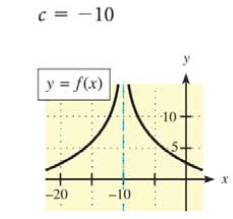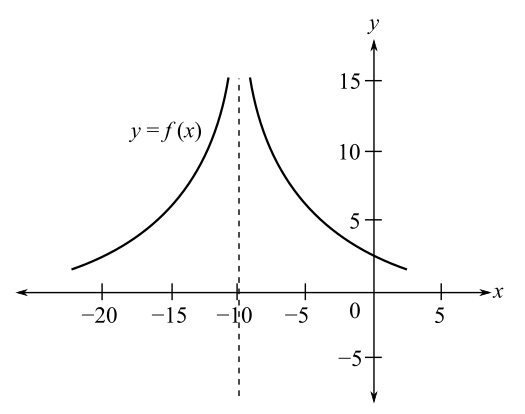Chapter 9.1, Problem 8EMathematical Applications for the ...

12th Edition
Ronald J. Harshbarger + 1 other
ISBN: 9781337625340

Solutions

Chapter
SectionMathematical Applications for the ...

12th Edition
Ronald J. Harshbarger + 1 other
ISBN: 9781337625340
Textbook Problem

In Problems 7-10, use the graph of y   =   f ( x ) and the given c-value to find the following, whenever they exist.(a) lim x → c − f ( x ) (b) lim x → c + f ( x ) (c) lim x → c f ( x ) (d) c = − 10(a)

To determine

The value of limxcf(x) by the use of the graph,Explanation

Given Information:

The value of c is 10. The graph of y=f(x) is,

Explanation:

Consider the provided graph,

The value of c is equal to 10.

The limit from the left is represented by limx10f(x)

(b)

To determine

The value of limxc+f(x) by the use of the graph,(c)

To determine

The value of limxcf(x) by the use of the graph,(d)

To determine

The value of f(c) by the use of the graph,Still sussing out bartleby?

Check out a sample textbook solution.

See a sample solution

The Solution to Your Study Problems

Bartleby provides explanations to thousands of textbook problems written by our experts, many with advanced degrees!

Get Started

In Exercises 2124, factor each expression completely. 21. 22r3 + 100r2

Applied Calculus for the Managerial, Life, and Social Sciences: A Brief Approach

Show that ddx(sin2x1+cotx+cos2x1+tanx)=cos2x

Single Variable Calculus: Early Transcendentals, Volume I

Which is the largest? a) f(a) b) f(b) c) f(c) d) cannot tell from information given

Study Guide for Stewart's Single Variable Calculus: Early Transcendentals, 8th

Graph each circle. x2+y2-8=0

College Algebra (MindTap Course List)

Define induction and deduction and explain the role of each in the scientific method.

Research Methods for the Behavioral Sciences (MindTap Course List)